• ## Boxplot

千次阅读 2019-02-23 14:07:09
In : import numpy as np import matplotlib.pyplot as plt In : data = np.random.randn(100) ...In : plt.boxplot(data) #The red bar is the median of the distribution. ...
In : import numpy as np
import matplotlib.pyplot as plt
In : data = np.random.randn(100)
In : plt.boxplot(data) #The red bar is the median of the distribution.
plt.show()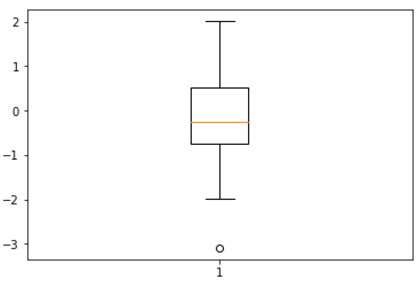​
In : import numpy as np
import matplotlib.pyplot as plt
In : data = np.random.randn(100,5)
In : plt.boxplot(data)
plt.show()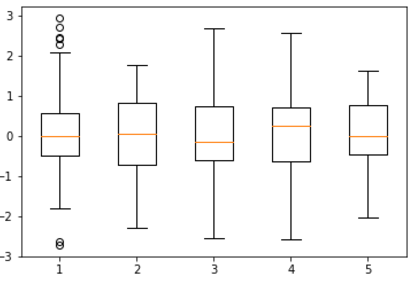​
5 Using custom colors for boxplots
In : import numpy as np
import matplotlib.pyplot as plt
In : values = np.random.randn(100)
b = plt.boxplot(values)      #Plotting functions returns a dictionary
#The key of the dictionary is the name of the graphical elements.
#such elements will be medians, fliers, whiskers, boxes, and caps.
#we iterate every graphic primitive that is a part of the boxplot and set its color to black.
for name, line_list in b.items():                   #b={name:key}
for line in line_list:
line.set_color('k') #Making a boxplot appear totally black
plt.show()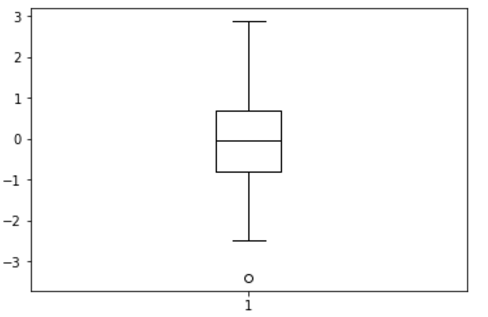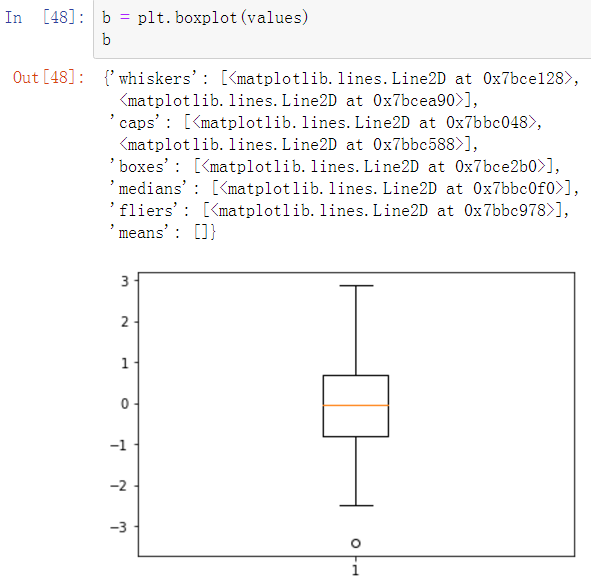Facet Grids分面网格 and Categorical Data类型数据
import seaborn as sns
import matplotlib.pyplot as plt
import numpy as np
import pandas as pd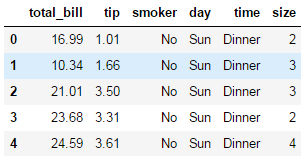tips['tip_pct'] = tips['tip'] / (tips['total_bill'] - tips['tip'])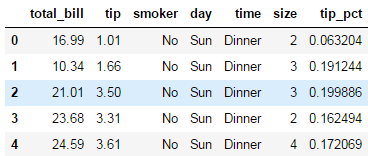#categorical data
sns.factorplot(x='day', y='tip_pct', hue='time', col='smoker', kind='bar', data=tips[tips.tip_pct <1])

plt.show()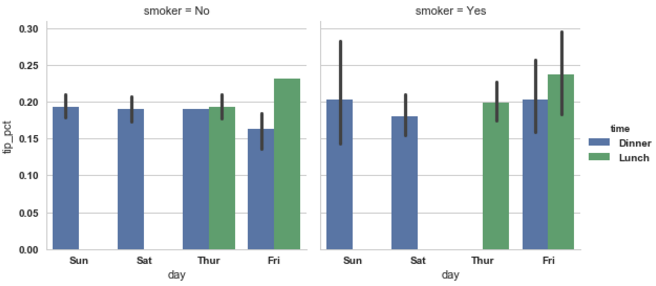#categorical data
sns.factorplot(x='day', y='tip_pct', row='time', col='smoker', kind='bar', data=tips[tips.tip_pct <1])

plt.show()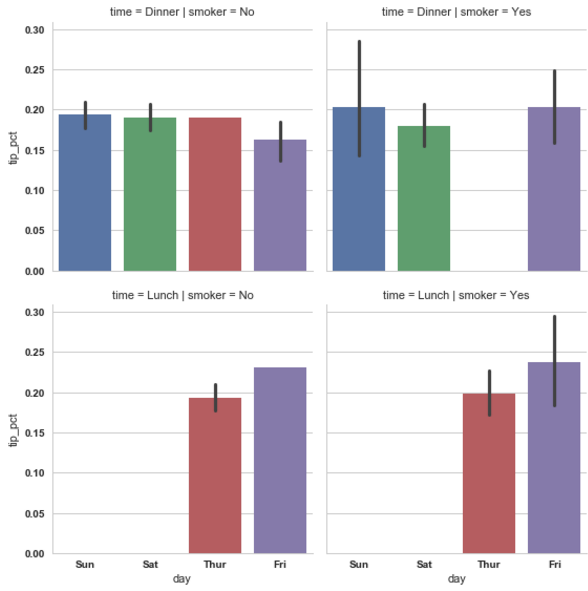sns.factorplot(x='tip_pct', y='day', kind='box', data=tips[tips.tip_pct<0.5])
plt.show()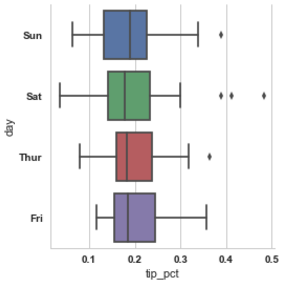展开全文• boxplot Definition : boxplot(x, notch=False, sym='b+', vert=True, whis=1.5, positions=None, widths=None, patch_artist=False, bootstrap=None, usermedians=None, conf_intervals=None, hold=None) Typ.....


boxplot
Definition : boxplot(x, notch=False, sym='b+', vert=True, whis=1.5, positions=None, widths=None, patch_artist=False, bootstrap=None, usermedians=None, conf_intervals=None, hold=None)

Type : Function of matplotlib.pyplot module

Make a box and whisker plot.

Call signature:

boxplot(x, notch=False, sym='+', vert=True, whis=1.5,
positions=None, widths=None, patch_artist=False,
bootstrap=None, usermedians=None, conf_intervals=None)
Make a box and whisker plot for each column of x or each vector in sequence x. The box extends from the lower to upper quartile values of the data, with a line at the median. The whiskers extend from the box to show the range of the data. Flier points are those past the end of the whiskers.

Function Arguments:

x :
Array or a sequence of vectors.
notch : [ False (default) | True ]
If False (default), produces a rectangular box plot. If True, will produce a notched box plot
sym : [ default ‘b+’ ]
The default symbol for flier points. Enter an empty string (‘’) if you don’t want to show fliers.
vert : [ False | True (default) ]
If True (default), makes the boxes vertical. If False, makes horizontal boxes.
whis : [ default 1.5 ]
Defines the length of the whiskers as a function of the inner quartile range. They extend to the most extreme data point within ( whis*(75%-25%) ) data range.
bootstrap : [ None (default) | integer ]
Specifies whether to bootstrap the confidence intervals around the median for notched boxplots. If bootstrap==None, no bootstrapping is performed, and notches are calculated using a Gaussian-based asymptotic approximation (see McGill, R., Tukey, J.W., and Larsen, W.A., 1978, and Kendall and Stuart, 1967). Otherwise, bootstrap specifies the number of times to bootstrap the median to determine it’s 95% confidence intervals. Values between 1000 and 10000 are recommended.
usermedians : [ default None ]
An array or sequence whose first dimension (or length) is compatible with x. This overrides the medians computed by matplotlib for each element of usermedians that is not None. When an element of usermedians == None, the median will be computed directly as normal.
conf_intervals : [ default None ]
Array or sequence whose first dimension (or length) is compatible with x and whose second dimension is 2. When the current element of conf_intervals is not None, the notch locations computed by matplotlib are overridden (assuming notch is True). When an element of conf_intervals is None, boxplot compute notches the method specified by the other kwargs (e.g., bootstrap).
positions : [ default 1,2,...,n ]
Sets the horizontal positions of the boxes. The ticks and limits are automatically set to match the positions.
widths : [ default 0.5 ]
Either a scalar or a vector and sets the width of each box. The default is 0.5, or 0.15*(distance between extreme positions) if that is smaller.
patch_artist : [ False (default) | True ]
If False produces boxes with the Line2D artist If True produces boxes with the Patch artist
Returns a dictionary mapping each component of the boxplot to a list of the matplotlib.lines.Line2D instances created. That dictionary has the following keys (assuming vertical boxplots):

boxes: the main body of the boxplot showing the quartiles and the median’s confidence intervals if enabled.
medians: horizonal lines at the median of each box.
whiskers: the vertical lines extending to the most extreme, n-outlier data points.
caps: the horizontal lines at the ends of the whiskers.
fliers: points representing data that extend beyone the whiskers (outliers).
Example:

Additional kwargs: hold = [True|False] overrides default hold state


以上为帮助说明，比较蛋疼的是http://matplotlib.org/api/pyplot_api.html， 官网上大量的参数都写得是不能用。是不是我的版本太低了。showXXXX等等。
简而言之：
x: list of lists.
notch: 豁口有无
sym: symbol, 异常值，不想要就赋成空字符。
vert: vertical
whis: 异常值设定。
bootstrap: 次数
usermedians: length等于list, 人为设定（是不是为数据造假准备的？）。
别的看重要再补上。

demo:
http://matplotlib.org/examples/pylab_examples/boxplot_demo2.html
http://matplotlib.org/examples/pylab_examples/boxplot_demo.html
http://blog.bharatbhole.com/creating-boxplots-with-matplotlib/
facecolor was aborted in the line2D class. If we wanted to chang the color in the box, we should use the Polygon class as demo 1.

转载于:https://www.cnblogs.com/hluo/p/4052460.html
展开全文•r语言
• 1.boxplot简化 2.boxplot对应点
1.boxplot简化2.boxplot对应点展开全文r语言
• <div><p>Hey, in the Series Boxplot you show the top 1k absolute values. However, may be some values which are fliers but don't have a big absolute value. In my opinion, you should take the ...
• ASCII箱图 ascii，仅用于字符的水平盒... var boxplot = require ( 'boxplot' ) boxplot ( { label1 : arrayOfSamples1 , label2 : arrayOfSamples2 , label3 : arrayOfSamples3 , } ) 原料药 boxplot(sourceData[,JavaScript
• 使用Angular的D3.js Boxplot指令（基于Mike Bostock的d3-boxplot和Gregory Hilkert的d3AngularIntegration） 请参阅： ： d3-boxplot 参见： : d3-angular集成 控制器： '\$ scope.selection'数组：箱线图下方的...JavaScript
• matlab开发-boxplot2。另一种箱线图函数
• 一、Boxplot 箱线图、 1、boxplot 函数、 2、代码示例、 二、Error Bar 误差条线图、 1、errorbar 函数、 2、代码示例、


文章目录
一、Boxplot 箱线图1、boxplot 函数2、代码示例
二、Error Bar 误差条线图1、errorbar 函数2、代码示例

一、Boxplot 箱线图

1、boxplot 函数

boxplot 函数文档 : https://ww2.mathworks.cn/help/stats/boxplot.html

stairs 函数语法 :
boxplot(x)
boxplot(x,g)

boxplot(x) : 根据 x 中的数据创建箱线图 ;
x 是向量 : 绘制一个箱子 ;x 是矩阵 : 为每个矩阵列绘制一个箱子 ;

箱子 :
中位数 : 每个箱子都有一个中心标记 , 表示中位数 ;第 25 百分位数 : 箱子底边 ;第 75 百分位数 : 箱子顶边 ;

boxplot(x,g) : g 中包含若干分组变量 ;

2、代码示例

代码示例 :
% 加载数据
% 不同国家中每加仑汽油能跑多少英里

% MPG 是箱线图数据
% Origin 中包含多个分组变量
boxplot(MPG, Origin);


绘图结果 :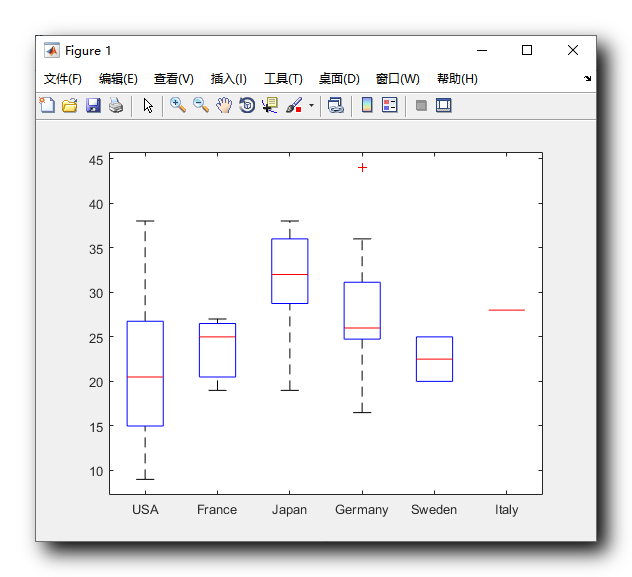二、Error Bar 误差条线图

1、errorbar 函数

errorbar 函数文档 : https://ww2.mathworks.cn/help/matlab/ref/errorbar.html

errorbar 函数语法 :
errorbar(y,err)
errorbar(x,y,err)

errorbar(y,err) 与 plot(y) 绘制的曲线相同 , err 参数指的是对应点的误差范围大小 ;
errorbar(x, y,err) 与 plot(x, y) 绘制的曲线相同 , err 参数指的是对应点的误差范围大小 ;

整个竖条 , 是有可能出现的误差数值 , errorbar 的第三个参数就是该误差数值的区间大小 ;

2、代码示例

代码示例 :
% 生成 x 向量, 0 ~ pi , 步长 pi / 10
x = 0 : pi / 10 : pi;

% 生成 y 轴的值对应向量
y = sin(x);

% 生成 e 向量 , 表示每个对应 x 位置的误差范围
e = std(y) * ones(size(x));

% 绘制含误差条的线图
% e 表示误差范围
errorbar(x, y, e);


绘图效果 :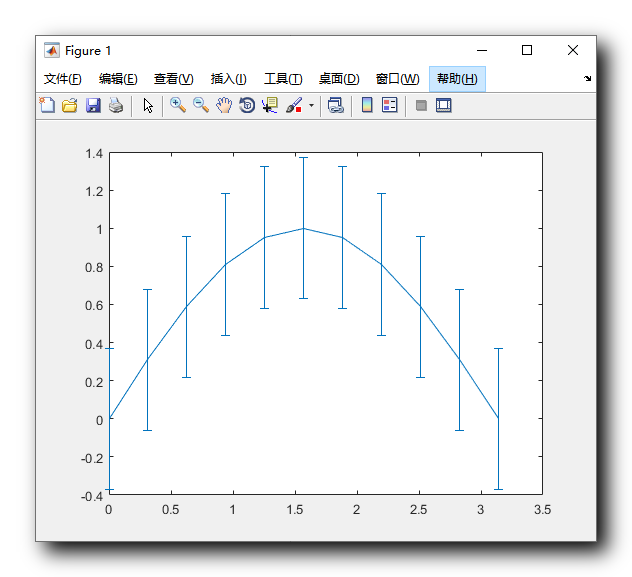展开全文matlab errorbar
• 然后我听说了一个用于倾斜数据的adjusted_boxplot，所以我也编码了其中一个，以及它使用的medcouple算法。 （底部引用） 我做了两个补充，可以改善调整后的箱形图的视觉外观。 它们在代码中进行了描述。 我认为这...matlab
• 首先，可以使用聚合计算组平均值：means 此数据集可与geom_text一起使用：library(ggplot2)ggplot(data=PlantGrowth, aes(x=group, y=weight, fill=group)) + geom_boxplot() +stat_summary(fun.y=mean, colour=...
• 问题：如何删除下图的“Boxplot grouped by path_num?” # 生成上图的代码 fig,ax=plt.subplots(figsize=(8,6)) df[df.path_num!=2].boxplot(column=['travel_time'],by='path_num',grid=False,ax=ax) plt.xlabel('...pandas
• ## boxplot analysis

千次阅读 2016-10-31 20:53:10
最近在摆弄数据离散度的时候遇到一种图形，叫做盒图(boxplot)。它对于显示数据的离散的分布情况效果不错。 盒图是在1977年由美国的统计学家约翰·图基(John Tukey)发明的。它由五个数值点组成：最小值(min)，下...
• EventCollection & Boxplot
• import { Boxplot , computeBoxplotStats } from 'react-boxplot' const values = [ 14 , 15 , 16 , 16 , 17 , 17 , 17 , 17 , 17 , 18 , 18 , 18 , 18 , 18 , 18 , 19 , 19 , 19 , 20 , 20 , ...
• <div><p>This class should handle the production of a matlibplot boxplot based on a ResultSet instance passed to its init method. <p>The functions to generate the boxes/whiskers, xticks, and title ...
• boxplot加显著性 library(ggplot2) library(ggsignif) head(iris) #自动添加显著性标记 compaired <- list(c(“versicolor”, “virginica”)) ggplot(iris, aes(x=Species, y=Sepal.Width, fill=Species)) + ......